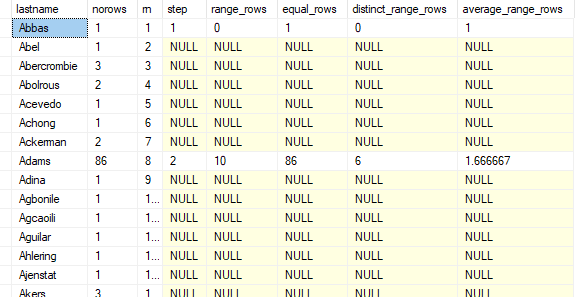# Inspecting the Stats Histogram with actual data data in table using Windows Functions

Hi,

I'm looking to compare the stats histogram on an index with the actual data distribution in the table to highlight exactly where that stats object is out of date. Struggling with windows functions to recreate range_rows totals to compare with the stats object. Hopefully below will be enough to explain what I'm trying to do...

-- *** add a bunch of data to person table (under stats refresh threshhold)

-- find the stats histogram for the on Person.Person.LastName
select s.name, c.Name
,'select * from sys.dm_db_stats_histogram (' + cast(s.object_id as varchar(30)) + ', '+cast(s.stats_id as varchar(10)) +'); ' ShowHistogram
from sys.stats s
left join sys.tables t
on s.object_id = t.object_id
left join sys.stats_columns sc
on s.object_id = sc.object_id
and s.stats_id = sc.stats_id
join sys.columns c
on sc.object_id = c.object_id
and sc.column_id = c.column_id
where t.name = 'person'
AND c.name = 'LastName'

-- inspect and highlight where data has been added in table vs the now out of date histogram

``````;with e as (
select lastname, count(*) norows, ROW_NUMBER() OVER (order by (select 1)) rn
from person.person
group by lastname
having count(*) >=1
)
select    e.lastname
, e.norows
, e.rn
, h.step_number step
, h.range_rows, h.equal_rows, h.distinct_range_rows, h.average_range_rows
from e
left join sys.dm_db_stats_histogram (274100017, 2) h
on e.LastName = h.range_high_key
ORDER BY rn``````

My problem is attempting to partition by the step on the null side. so perhaps I'll have to put results into temp table first and update the null rows to have a step number and then I'll be able to use the windows functions over the data set.

so where there is no row add one to include the step number.